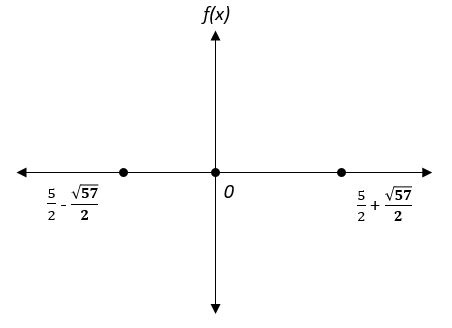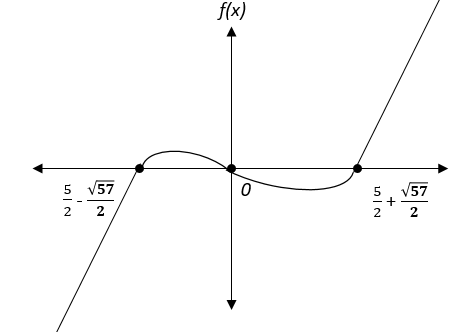# IPMAT Question Paper 2019 | IPM Indore Quants

###### IPMAT Sample Paper | IPMAT Question Paper | Question 39

IPMAT 2019 Question Paper IPM Indore Quantitative Ability. Solve questions from IPMAT 2019 Question Paper from IPM Indore and check the solutions to get adequate practice. The best way to ace IPMAT is by solving IPMAT Question Paper. To solve other IPMAT Sample papers, go here:IPM Sample Paper

Question 39: The function f(x) = $$frac{x^{3} - 5x^{2} - 8x}{3}\\$ is 1. positive and monotonically increasing for x $\in$-$infty, \frac{5-\sqrt{57}}{2}\\$) and x $\in$$frac{5+\sqrt{57}}{2}, +\infty\\$) 2. negative and monotonically decreasing for x $\in$-$infty, \frac{5-\sqrt{57}}{2}\\$ and x $\in$$frac{5+\sqrt{57}}{2},+\infty\\$) 3. negative and monotonically increasing for x $\in$-$infty, \frac{5-\sqrt{57}}{2}\\$) and positive and monotonically increasing for x $\in$$frac{5+\sqrt{57}}{2},+\infty\\$) 4. positive and monotonically increasing for x $\in$-$infty, \frac{5-\sqrt{57}}{2}\\$) and negative and monotonically decreasing for x $\in$$frac{5+\sqrt{57}}{2},+\infty\\$) ## Best CAT Online Coaching Try upto 40 hours for free Learn from the best! #### 2IIM : Best Online CAT Coaching. ### Video Explanation ## Best CAT Coaching in Chennai #### CAT Coaching in Chennai - CAT 2022Limited Seats Available - Register Now! ### Explanatory Answer Let’s find the roots of the function, That is where the function takes the value 0. f$x) = 0.
$$frac{x^{3} - 5x^{2} - 8x}{3}\\$ = 0 x3- 5x2- 8x = 0 x$x2−5x−8) = 0

x = 0 or x2−5x−8 = 0

x2−5x−8 = 0

x2−5x + $$frac{25}{4}\\$ −$\frac{25}{4}\\$ −8 = 0$x - $$frac{5}{2}\\$ )2- $\frac{57}{4}\\$ = 0$x - $$frac{5}{2}\\$ )2= $\frac{57}{4}\\$ x - $\frac{5}{2}\\$ = +$\frac{\sqrt{57}}{2}\\$$or) x - $$frac{5}{2}\\$ = - $\frac{\sqrt{57}}{2}\\$ x = $\frac{5}{2}\\$ + $\frac{\sqrt{57}}{2}\\$ or x = $\frac{5}{2}\\$ - $\frac{\sqrt{57}}{2}\\$ Therefore, the roots of the equation $\frac{x^{3} - 5x^{2} - 8x}{3}\\$ are 0, $\frac{5}{2}\\$ + $\frac{\sqrt{57}}{2}\\$, $\frac{5}{2}\\$ - $\frac{\sqrt{57}}{2}\\$ That means, when plotted f$x) will be 0 at 3 points when x = 0 or $$frac{5}{2}\\$ + $\frac{\sqrt{57}}{2}\\$ or $\frac{5}{2}\\$ - $\frac{\sqrt{57}}{2}\\$Arranged in increasing order the roots are $\frac{5}{2}\\$ - $\frac{\sqrt{57}}{2}\\$ , 0 , $\frac{5}{2}\\$ + $\frac{\sqrt{57}}{2}\\$. Check f$x) at a point which is less than $$frac{5}{2}\\$ - $\frac{\sqrt{57}}{2}\\$ and at a point greater than $\frac{5}{2}\\$ + $\frac{\sqrt{57}}{2}\\$ f$-2) = $$frac{$-2$^{3} - 5(-2)^{2} - 8(-2)}{3}$ = $\frac{-8-20-16}{3}\\$ = $\frac{-44}{3}\\$ = -ve. f$10) = $$frac{$10$^{3} - 5(10)^{2} - 8(10)}{3}$ = $\frac{1000-500-80}{3}\\$ = $\frac{420}{3}\\$ = 140 =+ve Since f$x) is negative below $$frac{5}{2}\\$ - $\frac{\sqrt{57}}{2}\\$ and positive above $\frac{5}{2}\\$ + $\frac{\sqrt{57}}{2}\\$. And since it is a continuous function, the function looks something like this.From the graph, only Option C is correct. f$x) is negative and monotonically increasing for x $$in$-$infty, \frac{5-\sqrt{57}}{2}\\$) and positive and monotonically increasing for x $\in$$frac{5+\sqrt{57}}{2},+\infty\\$) The question is"The function f$x) = $$frac{x^{3} - 5x^{2} - 8x}{3}\\$ is" ##### Hence, the answer is negative and monotonically increasing for x $\in$-$infty, \frac{5-\sqrt{57}}{2}\\$) and positive and monotonically increasing for x $\in$$frac{5+\sqrt{57}}{2},+\infty\\$) Choice C is the correct answer ###### Best Indore IPM & Rohtak IPM CoachingSignup and sample 9 full classes for free. Register now! ###### Already have an Account? ##### Where is 2IIM located? 2IIM Online CAT Coaching A Fermat Education Initiative, 58/16, Indira Gandhi Street, Kaveri Rangan Nagar, Saligramam, Chennai 600 093 ##### How to reach 2IIM? Mobile:$91) 99626 48484 / 94459 38484
WhatsApp: WhatsApp Now
Email: info@2iim.com